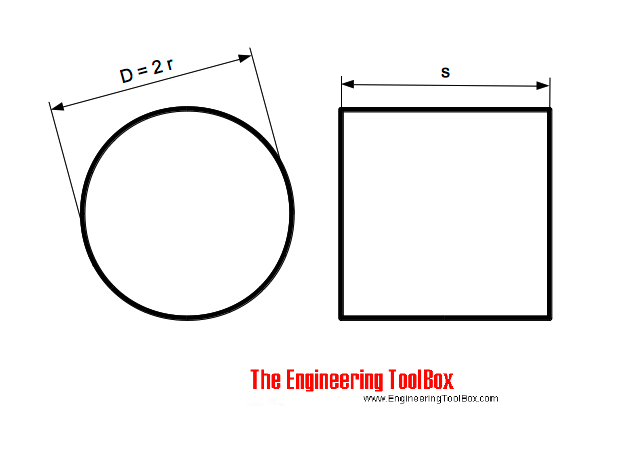Engineering ToolBox - Resources, Tools and Basic Information for Engineering and Design of Technical Applications!

# Equal Areas - Circles vs. Squares

## Radius and side lengths of equal areas, circles and squares.The area of a circle can be expressed as

Ac = π r2                             (1)

where

Ac = circle area (m2, ft2)

r = circle radius (m, ft)

The area of a square can be expressed as

As = s2                                 (2)

where

As = square area (m2, ft2)

s = side of square (m,ft)

If the area of the circle is equal to the area of the square - then Ac = As and (1) and (2) can be combined to

π r2 = s2                             (3)

### Radius of Circle - Side of Square is known

r = (s2/ π)1/2                              (4)

### Side of Square - Radius of a Circle if known

s = (π r2)1/2                               (5)

### Example - Circle and Square with equal Area

The side length of a square with the same area as a circle with radius 2 m can be calculated as

s = (π (2 m)2)1/2

= 3.54 m

## Related Topics

• Mathematics - Mathematical rules and laws - numbers, areas, volumes, exponents, trigonometric functions and more.

## Engineering ToolBox - SketchUp Extension - Online 3D modeling!

Add standard and customized parametric components - like flange beams, lumbers, piping, stairs and more - to your Sketchup model with the Engineering ToolBox - SketchUp Extension - enabled for use with the amazing, fun and free SketchUp Make and SketchUp Pro .Add the Engineering ToolBox extension to your SketchUp from the SketchUp Pro Sketchup Extension Warehouse!

Translate

## Privacy

We don't collect information from our users. Only emails and answers are saved in our archive. Cookies are only used in the browser to improve user experience.

Some of our calculators and applications let you save application data to your local computer. These applications will - due to browser restrictions - send data between your browser and our server. We don't save this data.

## Citation

• Engineering ToolBox, (2014). Equal Areas - Circles vs. Squares. [online] Available at: https://www.engineeringtoolbox.com/circle-square-area-d_1902.html [Accessed Day Mo. Year].

Modify access date.

. .

#### Scientific Online Calculator3 30